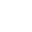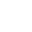# Multiplication Fluency is Achievable! Here’s How

If you haven’t read Part 1 and Part 2 of this series on multiplication fluency, here’s a recap.  The multiplication fluency strategies fall into two types:  foundational strategies and derivative strategies. The foundational strategies include learning the facts for the 2s, 5s, and 10s. Students also learn the Zero Property and Identity Properties of Multiplication (multiplying by 0 and 1).

In the final part of this three-part series on multiplication fluency, let’s explore the Derivative Strategies.  These are the strategies that are taught AFTER the student has demonstrated proficiency in the Foundation Strategies (see the previous post for an explanation of the Foundation Strategies).

The Derivative Strategies are the final steps that will eventually lead to multiplication fluency as well as, a better conceptual understanding of multiplication.

## Halving and Doubling for Multiplication Fluency

These are the strategies that continue to build that conceptual understanding of multiplication while building fluency over time.  The first strategy to teach is the halving and doubling strategy.  Why?

This strategy works with even factors.  So, if a student has learned and has fluency with the facts for the two times table, then he or she is ready to use this strategy to learn the facts for the four multiplication tables.

Watch this example of a student using the halving and doubling strategy.

As you can see, knowing the 2s helps with learning the 4s because two is half of four.  The same strategy can be applied to learning the 6 and 8 times table (though other strategies can be used as well).

We practice this strategy with paper and pencil first until students can start using the strategy using mental math.

## Adding a Group for Multiplication Fluency

This is the second strategy to teach students to use.  Once the 2s and 4s are learned by doubling and halving, students can move on to learning the three times table by learning to add a group.  If the student knows the 2s timetable, then teach the student to use this strategy to learn the 3s.

Watch this video of a student using the Add a Group Strategy.

As with all the strategies, we practice this strategy with paper and pencil first until students can start using the strategy using mental math.

## The Distributive Property Strategy

Of all the Properties of Multiplication, this is one of the hardest to teach.  But it is one of the most important in not only achieving multiplication fluency but will be needed later for more complex math like Algebra.

Conceptually, students need to understand decomposing a number, partial products, and writing equations.  Not an easy task. Obviously, students need to be explicitly taught the Distributive Property of Multiplication.

But whatever resources you use, once students are familiar with it, they can then practice the strategy for multiplication fluency for seven and eight.

Before teaching this strategy, students really should be proficient in the facts for five.  Why? It is easier to decompose a number when one of the factors is five.  Multiplying by five leads to products with zero and five, which when added to the other partial product is easier to do mentally.

Watch this video of a student using the Distributive Property of Multiplication.

There’s a lot involved in using this multiplication property, so using paper and pencil to practice first is a must.

## Other Derivative Strategies

There are several other strategies to teach which include subtracting a group, using a nearby square, and using the Commutative Property of Multiplication.

There is a definite sequence of teaching the multiplication tables.  These strategies can not be learned in a day or a week. Just like the strategies for mental math for addition took months or even years to internalize, so will these. But imagine how efficient students will become when they learn to use these multiplication fluency strategies!

Sign up for my newsletter and receive the FREE Guide to Achieving Multiplication Fluency. It’s a 10-page guide that explains all of these strategies. It also offers some suggestions on how to teach each strategy as well as 2 games to practice the strategies! Just sign up below!

If you have any questions about any of the strategies, just pop in a question below in the comments.

## Save Time and Get Started Right Away!

If you’re like most teachers, time is a precious commodity of which we have very little of! So, I’ve created a resource that has everything you need to teach these strategies over time.

The Multiplication Fluency Strategies Resources includes teaching posters, student templates, practice pages, and games. See below for examples of each!

These are just a few of the 38 color charts available in the full resource.

Also included:

• A Sample Lesson Plan for a Foundation Strategy
• A Sample Lesson Plan for a Derivative Strategy
• Sequence for teaching the multiplication tables aligned with strategies
• Support Printables for the Foundation Strategies that include games, flashcards, bookmarks and more
• Support Printables for the Derivative Strategies that include games and templates

These games can be used in centers, small groups or the entire class. They are low prep: just print, add some game pieces and a penny or paper clip and you’re ready to play!

These templates are used for practicing the strategy.  Just print and laminate them for use over and over until the students can internalize the strategy.

The practice pages can be used as independent work, put in centers, small group intervention or even homework.

I’ve also included sample lesson plans for the Foundation Strategies and Derivative Strategies.  It also includes the teaching sequence for the multiplication tables and well as, explanations for each of the strategies.

It’s a resource that can be used in a wide variety of settings:  whole group, small group, intervention group, guided math groups or even at home with your own children. You’ll have everything you need to help your students or child achieve multiplication fluency.

## Looking for More Multiplication Ideas, Tips, and Lessons? Check out the articles below!

Distributive Property of Multiplication – How to Break it Down

Learning Multiplication is More than Memorization!

Multiplication Practice Made Fun and Easy

## Don’t Go Yet!

Are you new to the 1:1 classroom setting? Then you’ll want to read my Valuable Tips for the 1:1 Classroom.

Check out how I use Google Classroom to present at Back to School Night for Parents.

Or register for the newsletter to receive this FREE Guide to Achieving Multiplication Fluency. Get it now by signing up for my newsletter below!###### Follow:

I’m a single dad raising two boys, 15 and 12. I’m also a veteran teacher of over 30 years who has taught first, second and third grades. I’m also a trained literacy coach, served as a mentor teacher and have a Bilingual Specialist Credential. I’m currently serving as a District Math Coach.

Share:

### Interactive Digital Notebooks for Google Slides®